# FINAL CONTROL ELEMENT FINAL CONTROL ELEMENT The final

• Slides: 76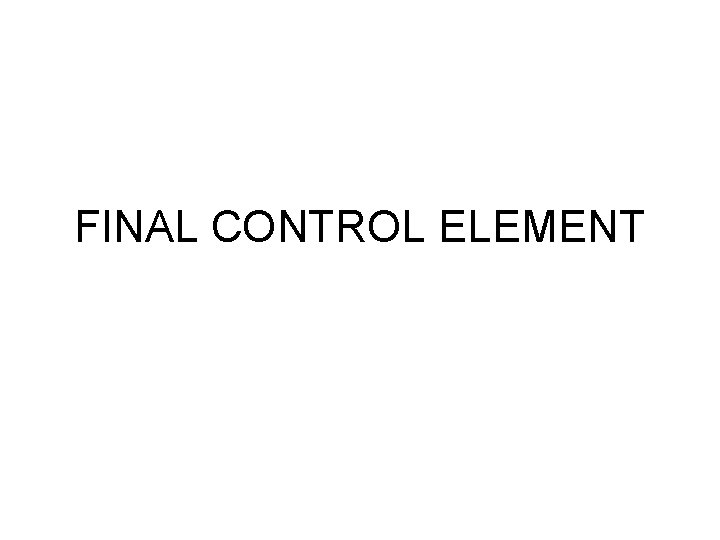FINAL CONTROL ELEMENT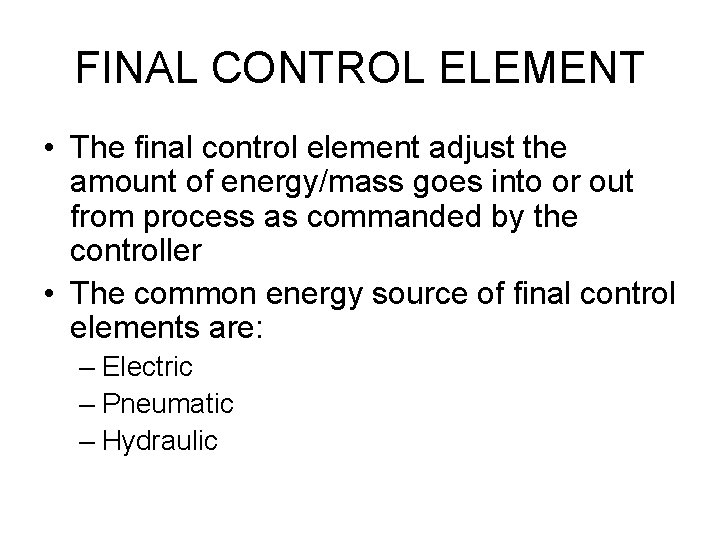FINAL CONTROL ELEMENT • The final control element adjust the amount of energy/mass goes into or out from process as commanded by the controller • The common energy source of final control elements are: – Electric – Pneumatic – HydraulicELECTRIC FINAL CONTROL ELEMENT • • • Electric current/voltage Solenoid Stepping Motor DC Motor AC Motor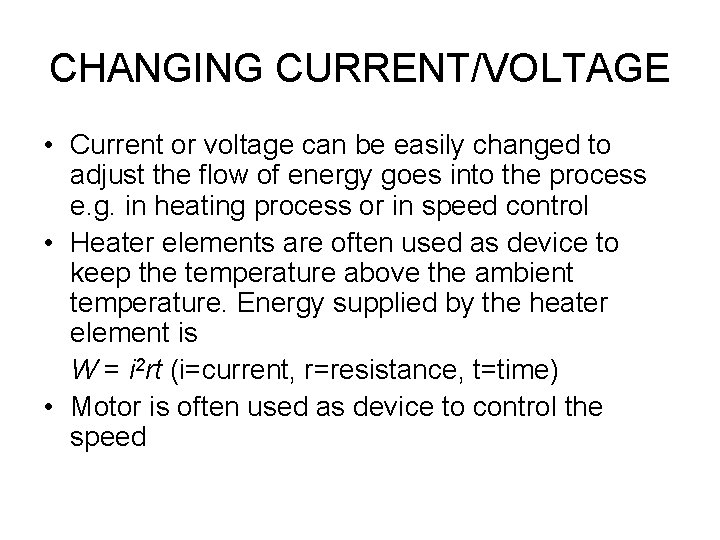CHANGING CURRENT/VOLTAGE • Current or voltage can be easily changed to adjust the flow of energy goes into the process e. g. in heating process or in speed control • Heater elements are often used as device to keep the temperature above the ambient temperature. Energy supplied by the heater element is W = i 2 rt (i=current, r=resistance, t=time) • Motor is often used as device to control the speedCHANGING CURRENT/VOLTAGE • Using – Potentiometer – Amplifier – Ward Leonard system – Switch (on-off action)Changing Current/Voltage Using Rheostat I = V/(R 1+R 2) Rheostat Heater R 1 Power at rheostat P 1 =I 2 R 1 V R 2 Power at heater P 2 =I 2 R 2 I Disadvantage loss of power at rheostatExample of Heating elements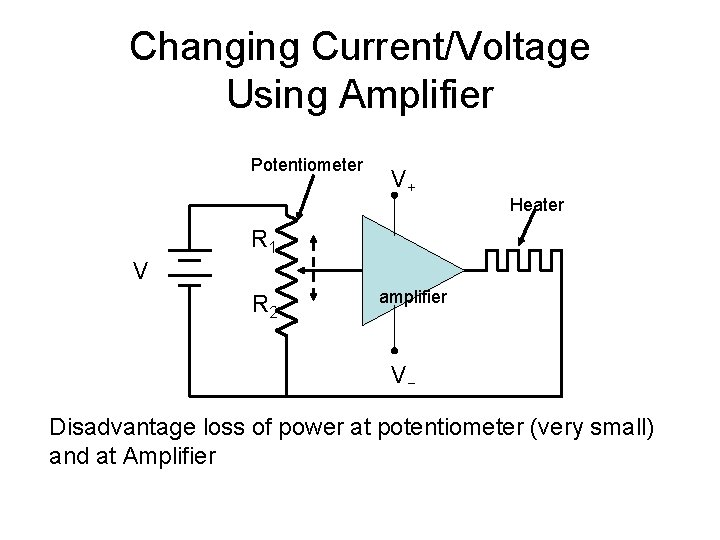Changing Current/Voltage Using Amplifier Potentiometer V+ Heater R 1 V R 2 amplifier V− Disadvantage loss of power at potentiometer (very small) and at Amplifier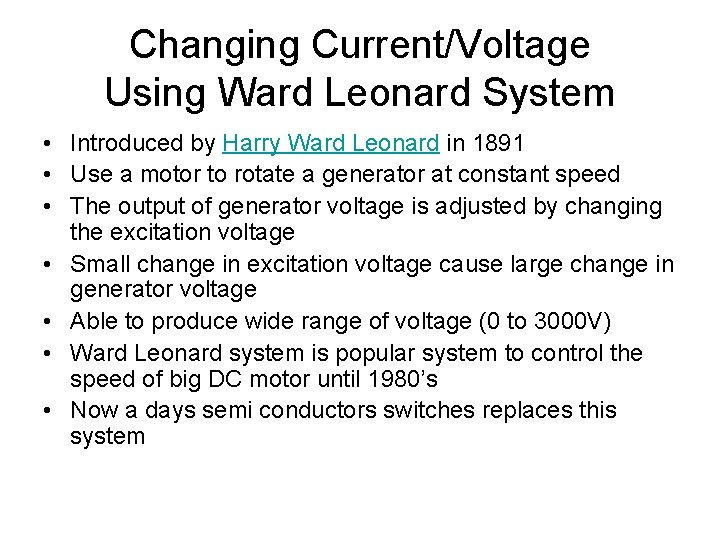Changing Current/Voltage Using Ward Leonard System • Introduced by Harry Ward Leonard in 1891 • Use a motor to rotate a generator at constant speed • The output of generator voltage is adjusted by changing the excitation voltage • Small change in excitation voltage cause large change in generator voltage • Able to produce wide range of voltage (0 to 3000 V) • Ward Leonard system is popular system to control the speed of big DC motor until 1980’s • Now a days semi conductors switches replaces this system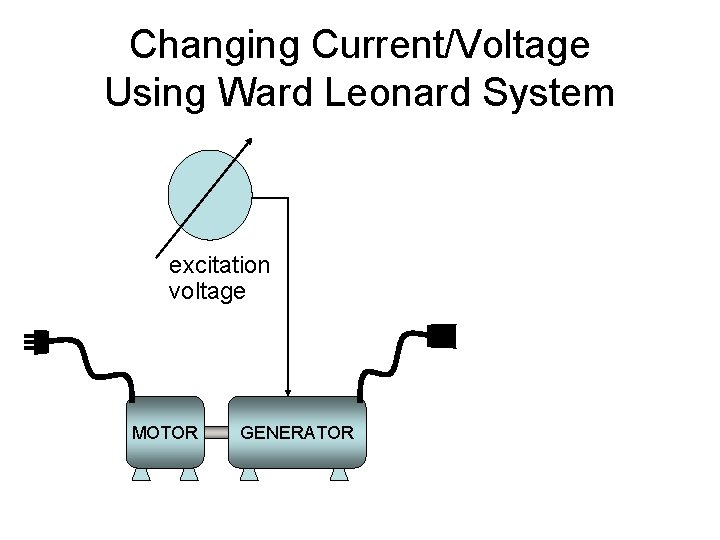Changing Current/Voltage Using Ward Leonard System excitation voltage MOTOR GENERATOR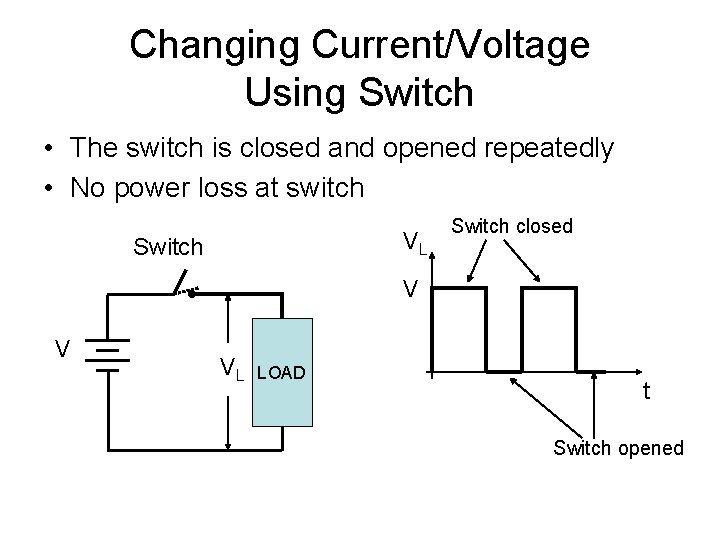Changing Current/Voltage Using Switch • The switch is closed and opened repeatedly • No power loss at switch VL Switch closed V V VL LOAD t Switch opened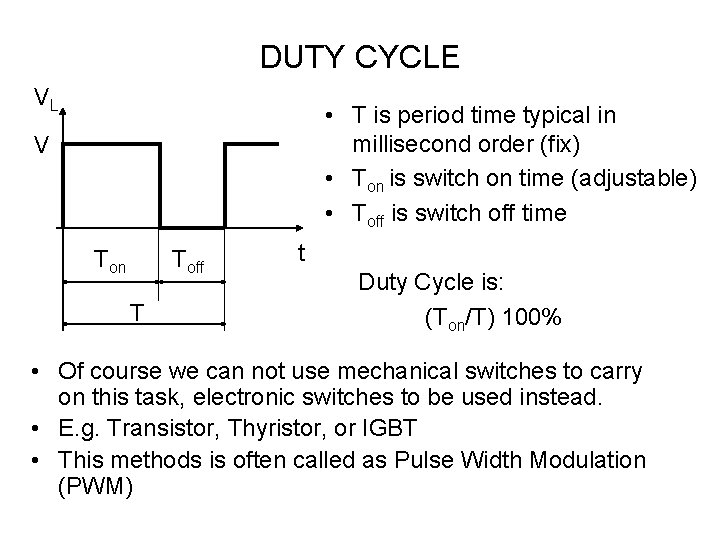DUTY CYCLE VL • T is period time typical in millisecond order (fix) • Ton is switch on time (adjustable) • Toff is switch off time V Ton Toff T t Duty Cycle is: (Ton/T) 100% • Of course we can not use mechanical switches to carry on this task, electronic switches to be used instead. • E. g. Transistor, Thyristor, or IGBT • This methods is often called as Pulse Width Modulation (PWM)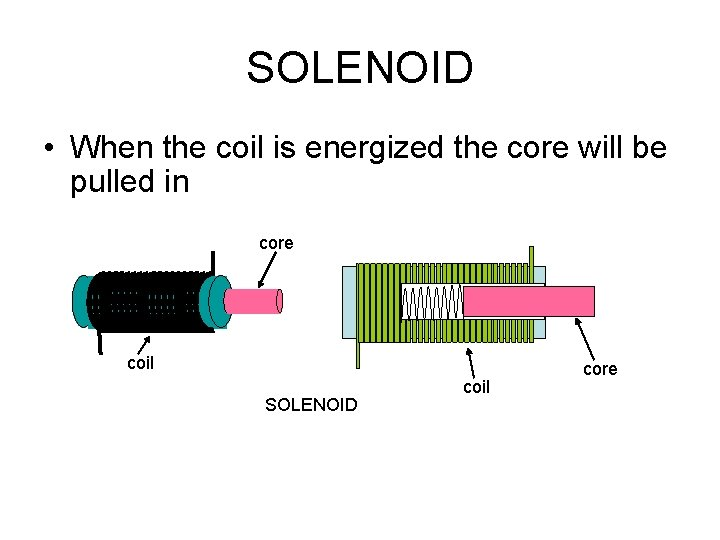SOLENOID • When the coil is energized the core will be pulled in core coil SOLENOID coil core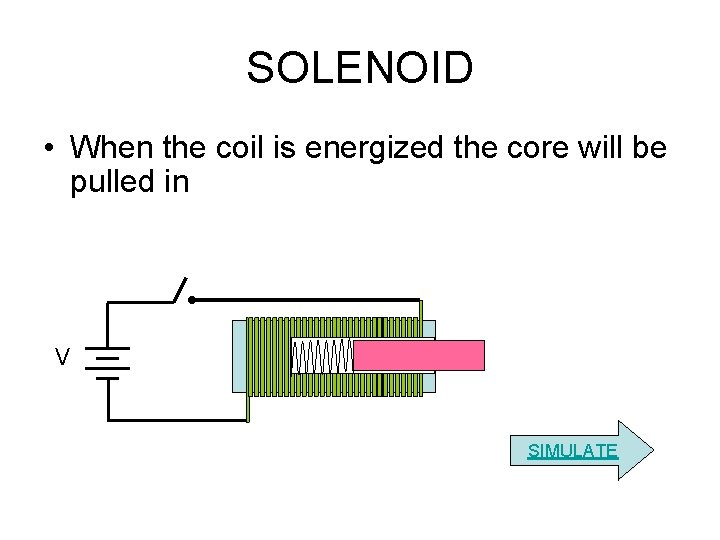SOLENOID • When the coil is energized the core will be pulled in V SIMULATE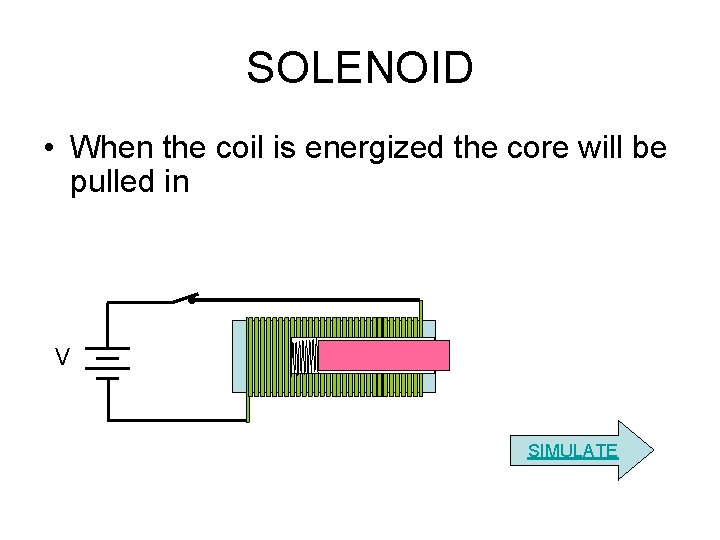SOLENOID • When the coil is energized the core will be pulled in V SIMULATE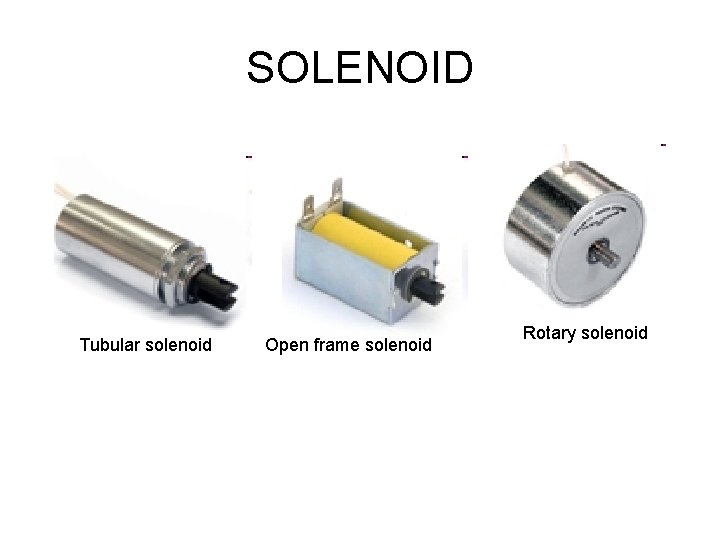SOLENOID Tubular solenoid Open frame solenoid Rotary solenoid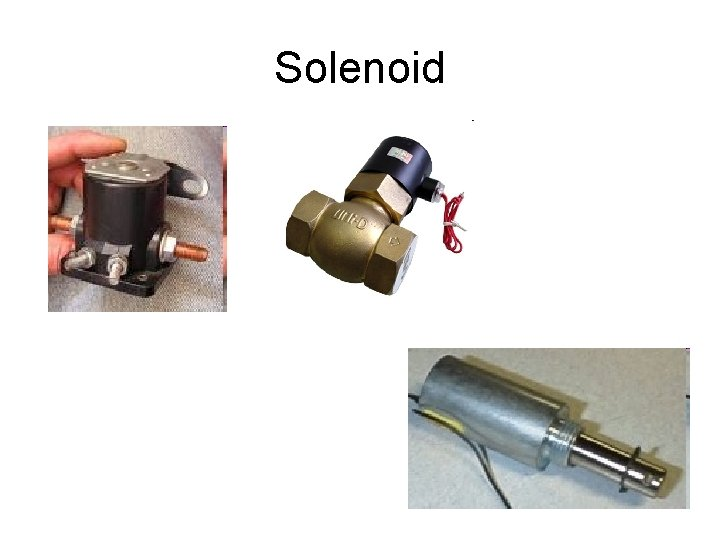SolenoidSolenoid Usage • • • pushing buttons, hitting keys on a piano, Open closed Valve, Heavy duty contactor jumping robots etcSTEPPING MOTOR The top electromagnet (1) is turned on, attracting the nearest teeth of a gear-shaped iron rotor. With the teeth aligned to electromagnet 1, they will be slightly offset from electromagnet The top electromagnet (1) is turned off, and the right electromagnet (2) is energized, pulling the nearest teeth slightly to the right. This results in a rotation of 3. 6° in this example.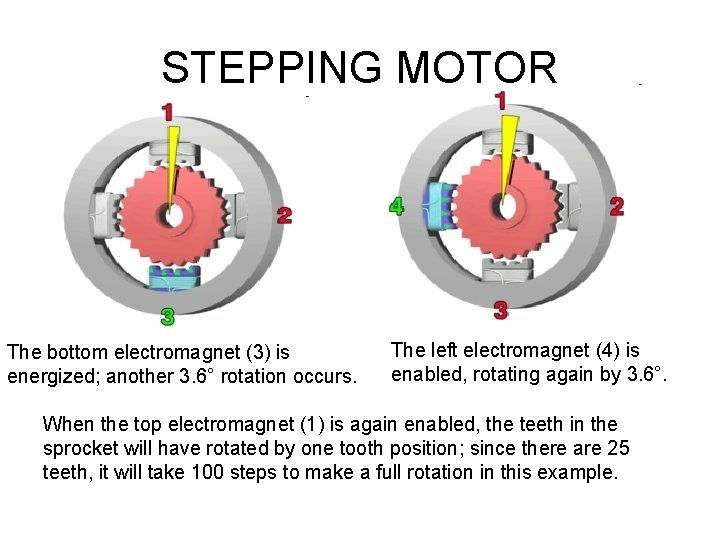STEPPING MOTOR The bottom electromagnet (3) is energized; another 3. 6° rotation occurs. The left electromagnet (4) is enabled, rotating again by 3. 6°. When the top electromagnet (1) is again enabled, the teeth in the sprocket will have rotated by one tooth position; since there are 25 teeth, it will take 100 steps to make a full rotation in this example.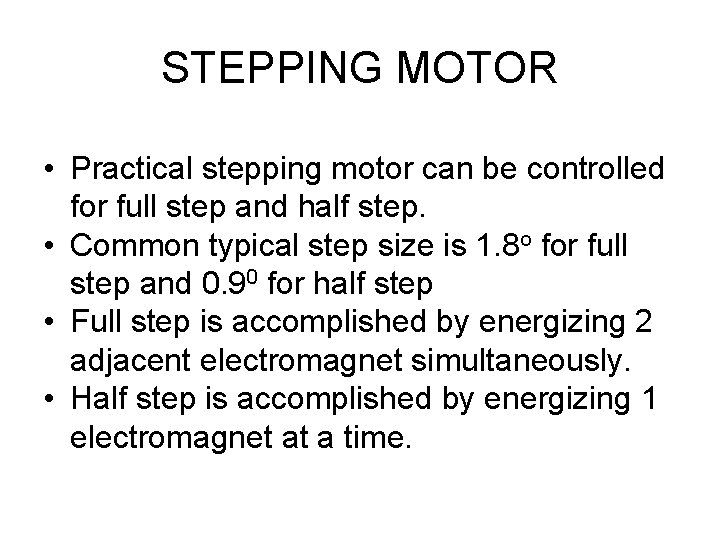STEPPING MOTOR • Practical stepping motor can be controlled for full step and half step. • Common typical step size is 1. 8 o for full step and 0. 90 for half step • Full step is accomplished by energizing 2 adjacent electromagnet simultaneously. • Half step is accomplished by energizing 1 electromagnet at a time.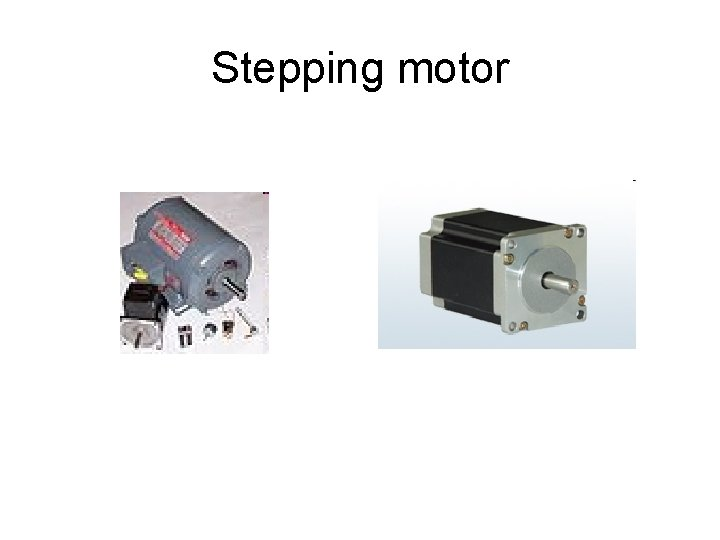Stepping motor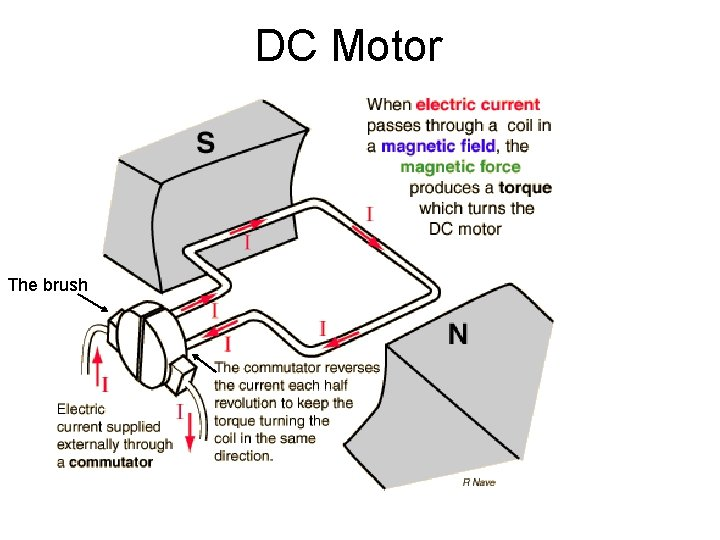DC Motor The brush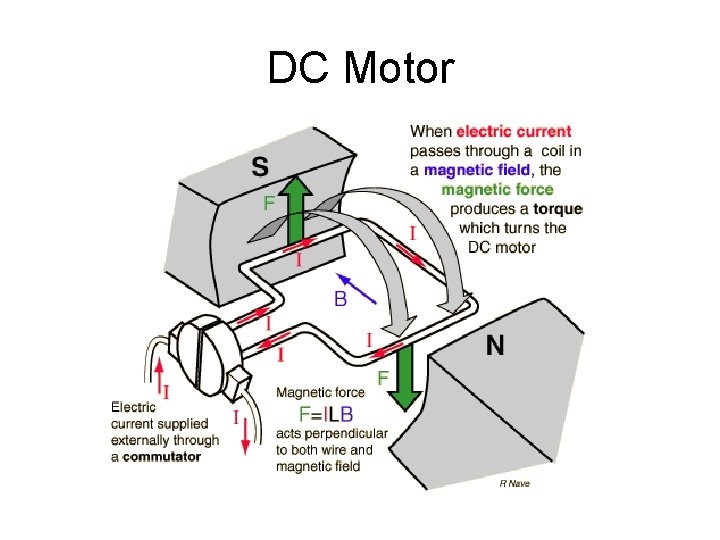DC MotorPractical DC Motors Every DC motor has six basic parts – axle, rotor (a. k. a. , armature), stator, commutator, field magnet(s), For a small motor the magnets is made from permanent magnet and brushes.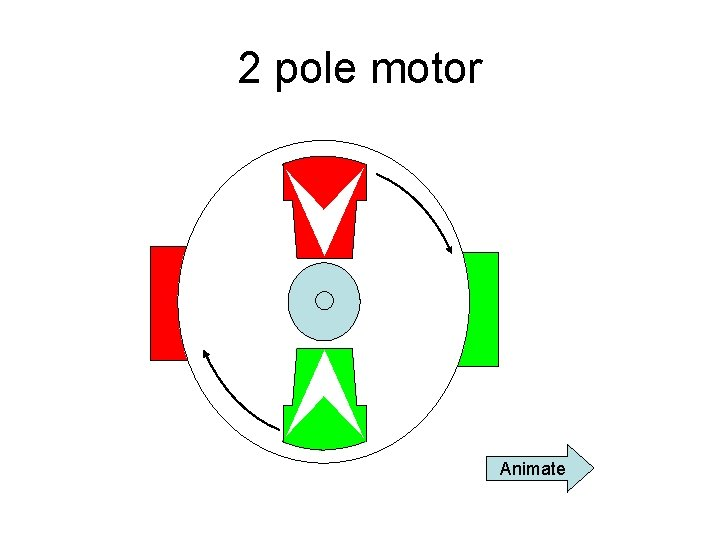2 pole motor Animate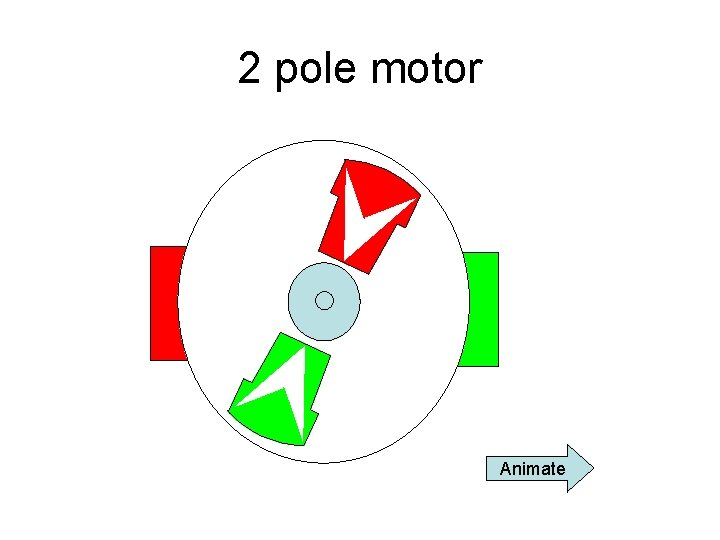2 pole motor Animate2 pole motor Animate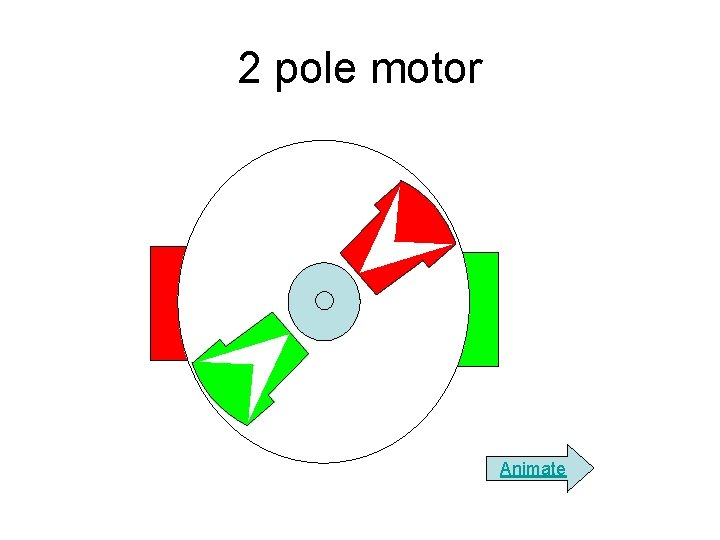2 pole motor Animate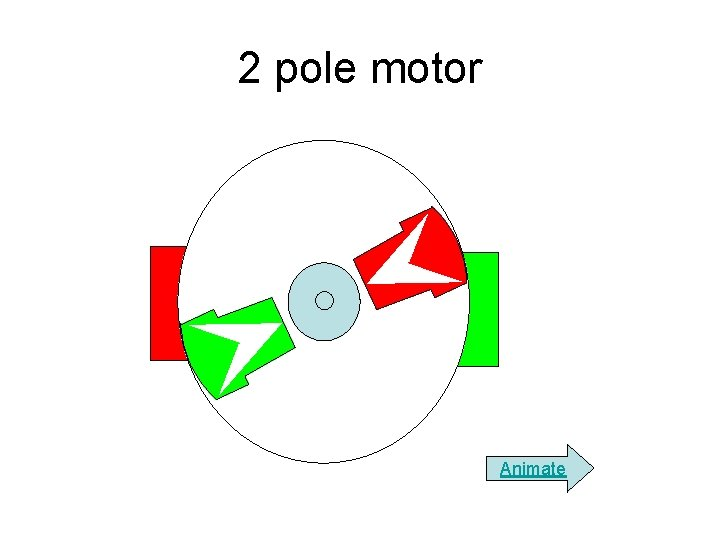2 pole motor Animate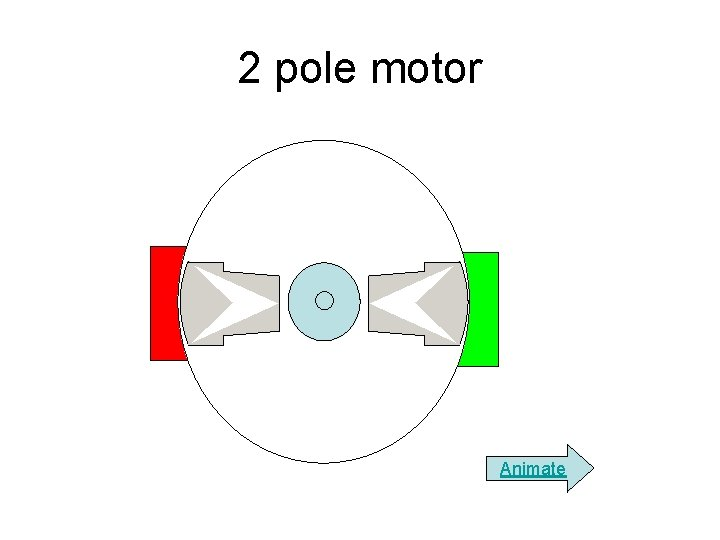2 pole motor Animate2 pole motor Animate2 pole motor Animate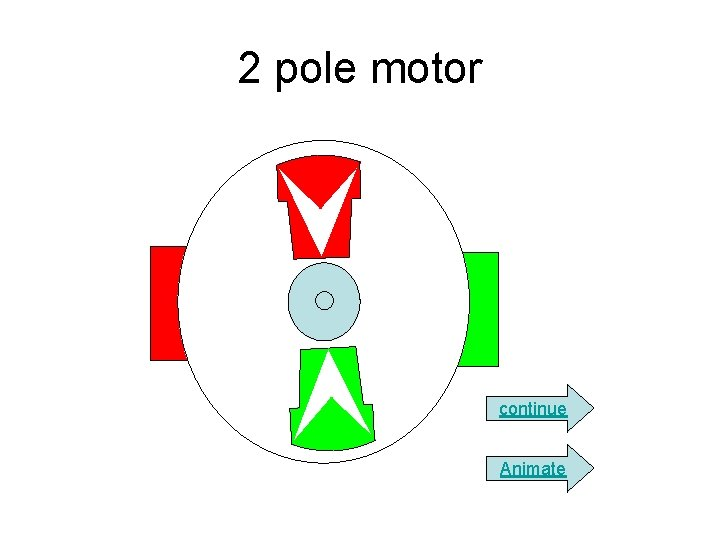2 pole motor continue Animate3 pole DC motors 1 The coil for each poles are connected serially. 2 3 + − The commutator consist of 3 sector, consequently one coil will be fully energized and the others will be partially energized.3 pole DC motors The commutator and the coil is arranged in such a way that the polarity of each pole is as shown animate next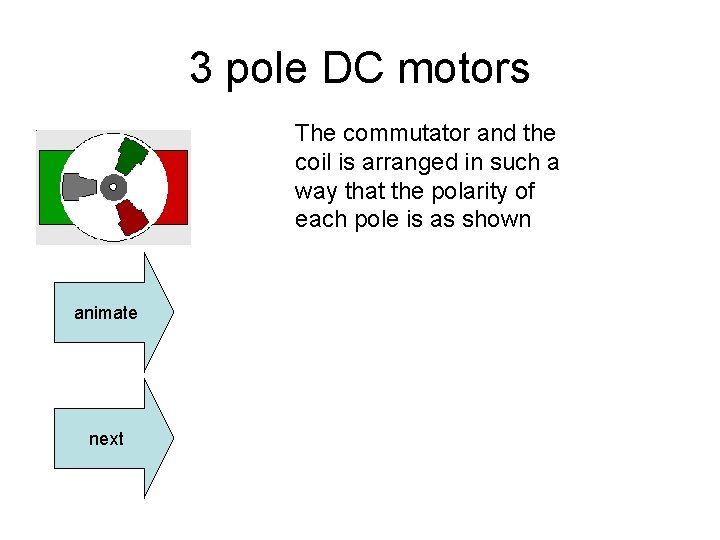3 pole DC motors The commutator and the coil is arranged in such a way that the polarity of each pole is as shown animate next3 pole DC motors The commutator and the coil is arranged in such a way that the polarity of each pole is as shown animate next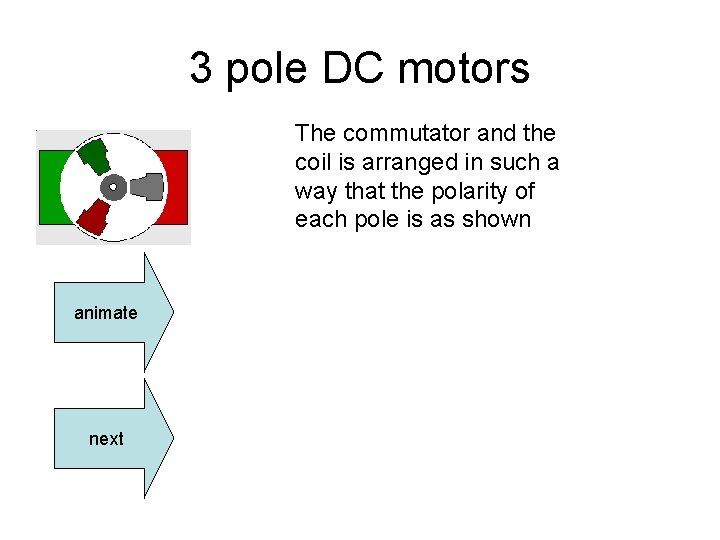3 pole DC motors The commutator and the coil is arranged in such a way that the polarity of each pole is as shown animate nextDC motors • As the rotor is rotating, back emf (Ea) will be produced, the faster the rotor turn the higher Ea and the smaller Ia. V • The starting current of motors will be much higher then the rating current. Ia Ea motorDC motors Field winding Armature winding For big motors the magnet is made from coil and core. The current flowing in the coil is called If and the current flowing in the armature is called Ia. The armature winding and the field winding are connected to a common power supply The armature winding and the field winding are often connected in series, parallel, or compound. The torque characteristic will be different for each connection. The figure shows a parallel connection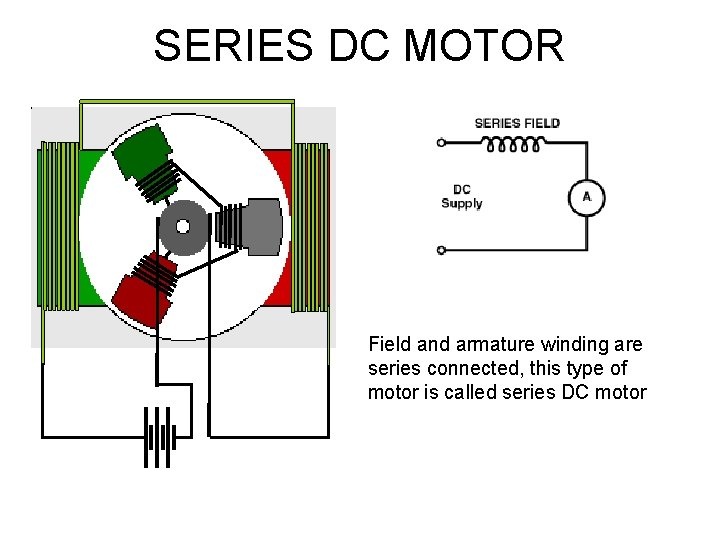SERIES DC MOTOR Field and armature winding are series connected, this type of motor is called series DC motor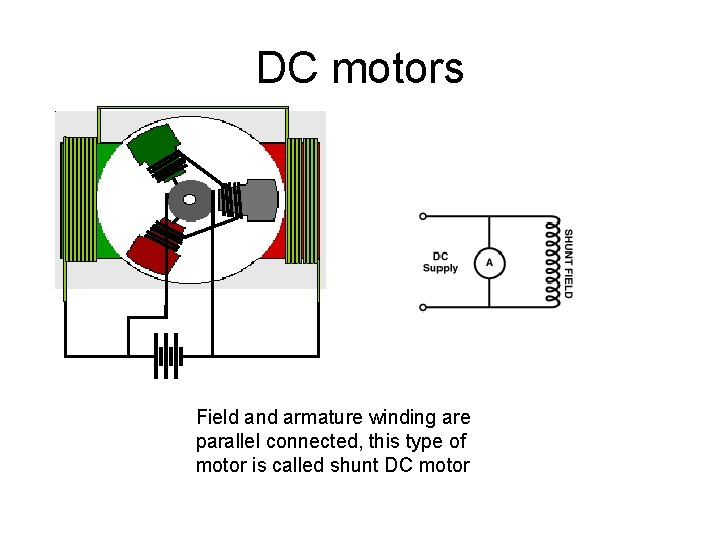DC motors Field and armature winding are parallel connected, this type of motor is called shunt DC motor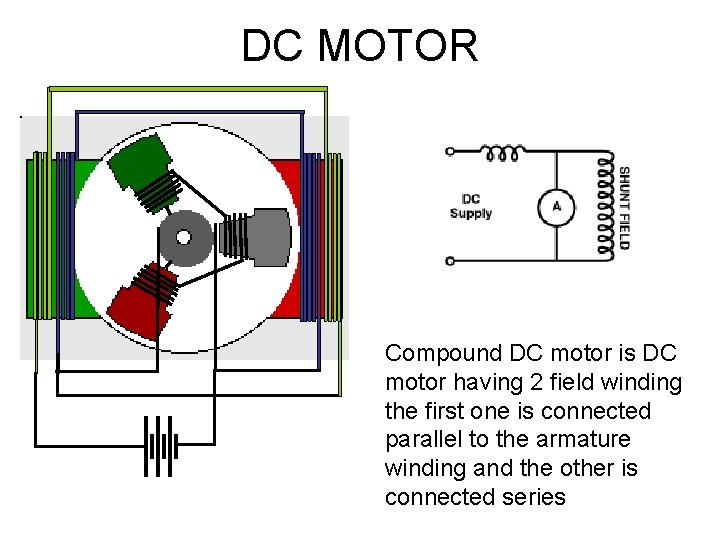DC MOTOR Compound DC motor is DC motor having 2 field winding the first one is connected parallel to the armature winding and the other is connected seriesDC MOTOR Torque: T = KΦIa – K is a constant – Φ magnetic flux – Ia is armature current • Magnetic flux is constant if it is from permanent magnet • It is depend on the If if it is produced by currentDC MOTOR TORQUE-SPEED CURVE Torque: T = KΦIaSERIES DC MOTOR TORQUESPEED CURVE Torque: T = KΦIa T= KIa 2SHUNT DC MOTOR TORQUESPEED CURVE Torque: T = KΦIa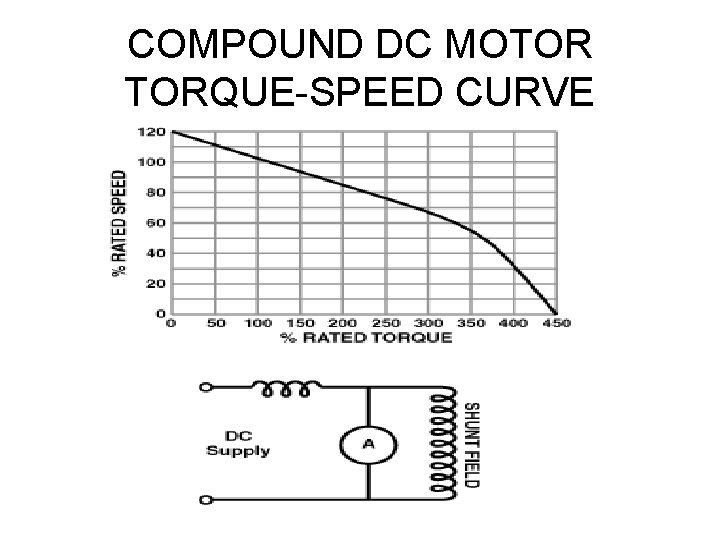COMPOUND DC MOTOR TORQUE-SPEED CURVE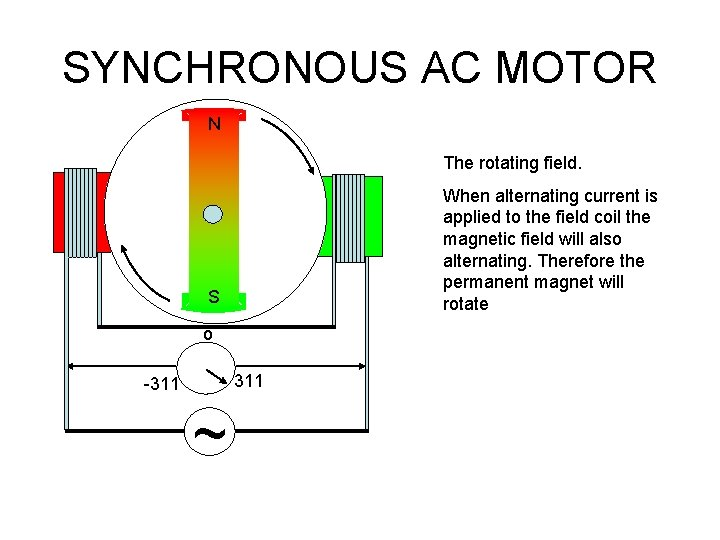SYNCHRONOUS AC MOTOR N The rotating field. When alternating current is applied to the field coil the magnetic field will also alternating. Therefore the permanent magnet will rotate S o 311 -311 ~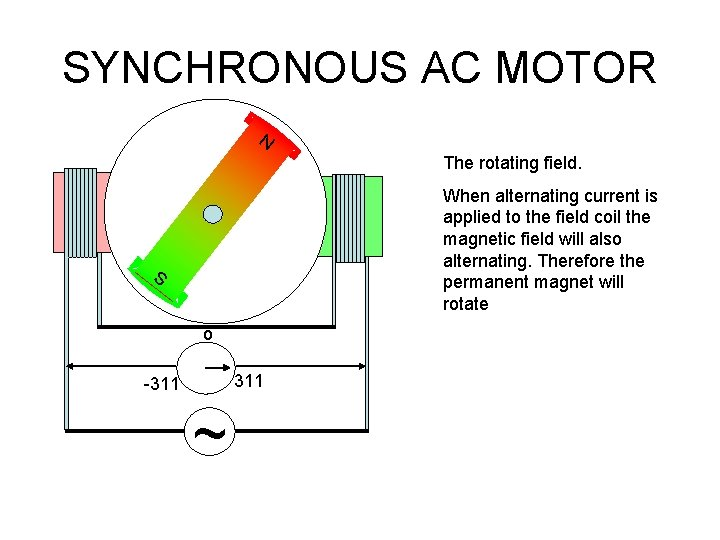SYNCHRONOUS AC MOTOR N The rotating field. When alternating current is applied to the field coil the magnetic field will also alternating. Therefore the permanent magnet will rotate S o 311 -311 ~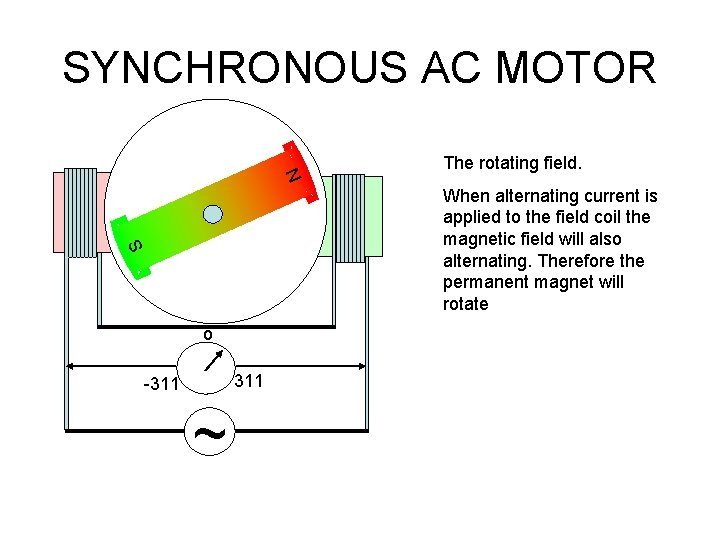SYNCHRONOUS AC MOTOR N The rotating field. S When alternating current is applied to the field coil the magnetic field will also alternating. Therefore the permanent magnet will rotate o 311 -311 ~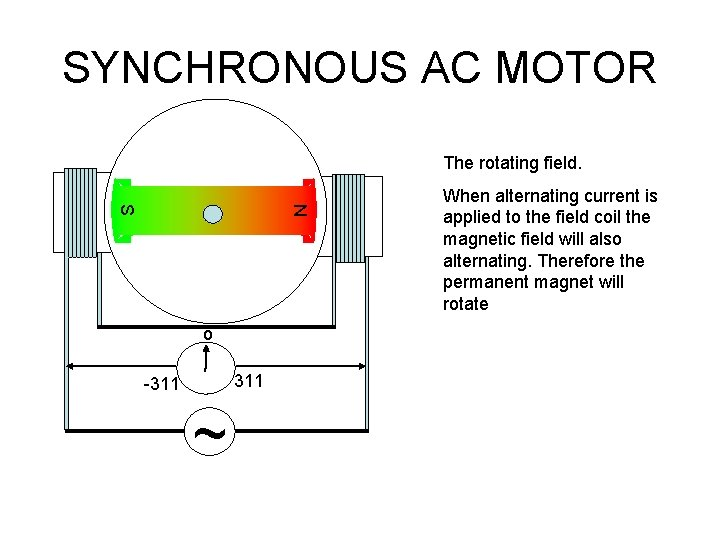SYNCHRONOUS AC MOTOR The rotating field. N S o 311 -311 ~ When alternating current is applied to the field coil the magnetic field will also alternating. Therefore the permanent magnet will rotate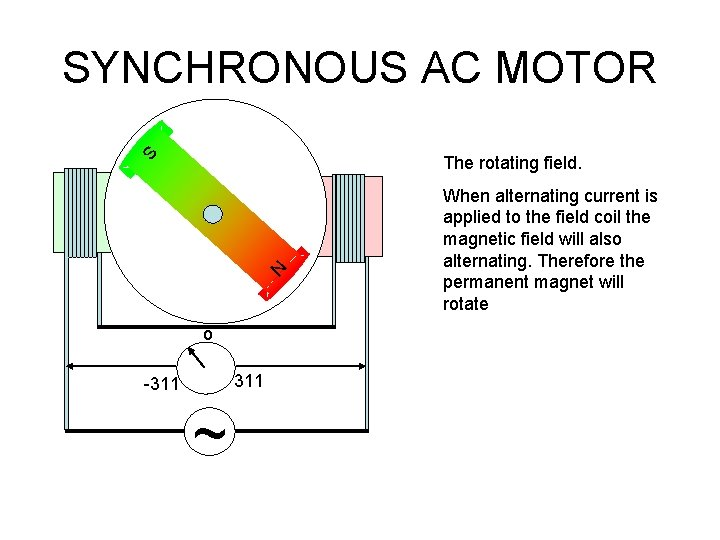SYNCHRONOUS AC MOTOR S The rotating field. N o 311 -311 ~ When alternating current is applied to the field coil the magnetic field will also alternating. Therefore the permanent magnet will rotate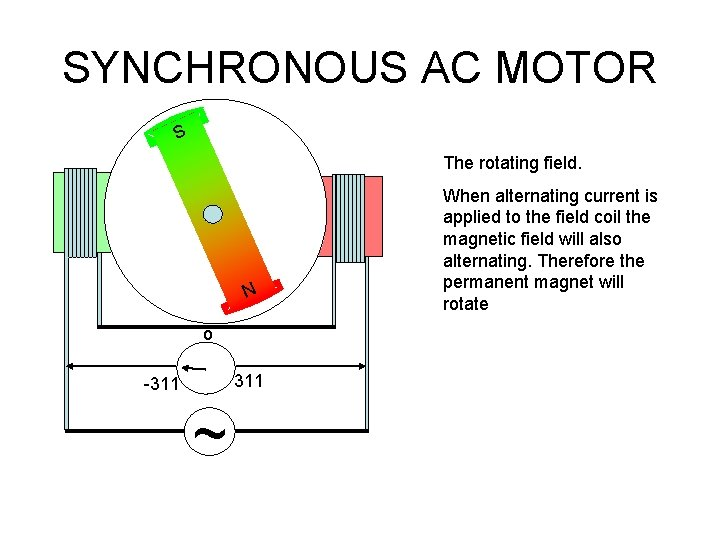SYNCHRONOUS AC MOTOR S The rotating field. N o 311 -311 ~ When alternating current is applied to the field coil the magnetic field will also alternating. Therefore the permanent magnet will rotateSYNCHRONOUS AC MOTOR S This motor has 2 poles If the frequency of the current is f hertz (cycle/s) then the rpm n = f rps N n = (120 f)/p rpm o 311 -311 ~ Where p is the number of the polesSYNCHRONOUS AC MOTOR S N 4 pole motorTHREE PHASE SYNCHRONOUS AC MOTOR R S T S R S T N 4 pole 3Φ motor R S T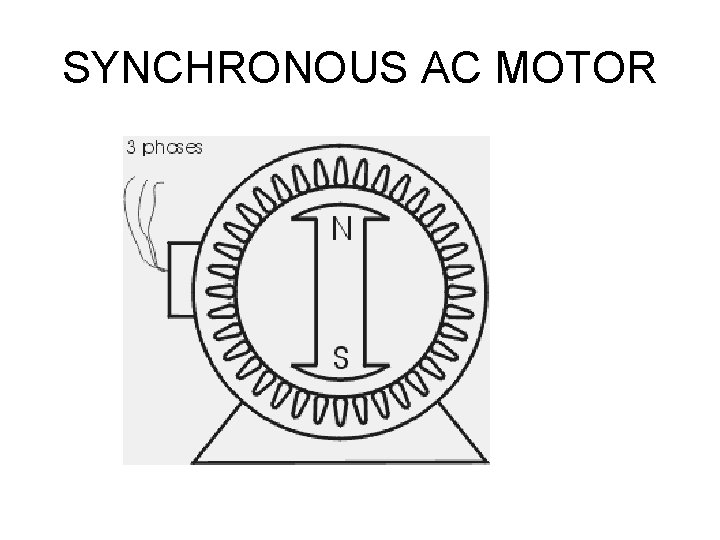SYNCHRONOUS AC MOTOR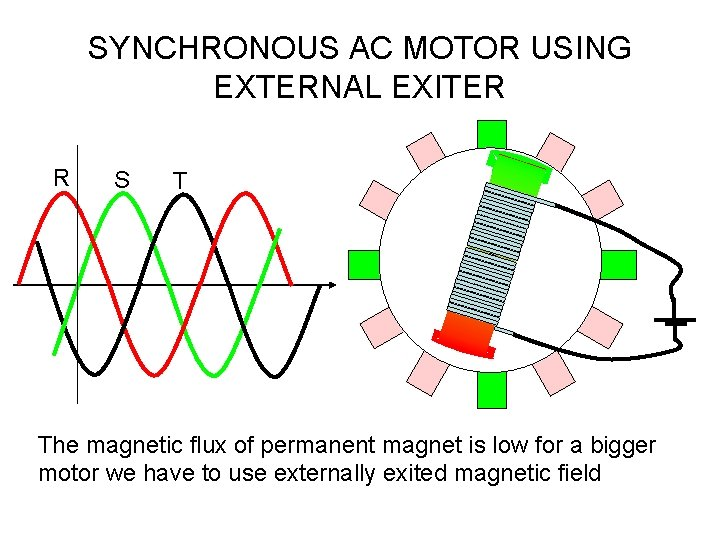SYNCHRONOUS AC MOTOR USING EXTERNAL EXITER R S T The magnetic flux of permanent magnet is low for a bigger motor we have to use externally exited magnetic field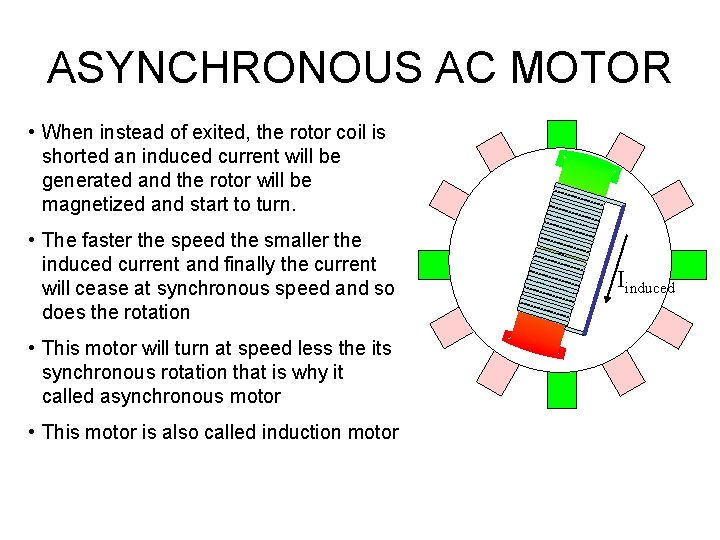ASYNCHRONOUS AC MOTOR • When instead of exited, the rotor coil is shorted an induced current will be generated and the rotor will be magnetized and start to turn. • The faster the speed the smaller the induced current and finally the current will cease at synchronous speed and so does the rotation • This motor will turn at speed less the its synchronous rotation that is why it called asynchronous motor • This motor is also called induction motor IinducedCalculating Motor Speed • A squirrel cage induction motor is a constant speed device. It cannot operate for any length of time at speeds below those shown on the nameplate without danger of burning out. • To Calculate the speed of a induction motor, apply this formula: Srpm = 120 x F P Srpm = synchronous revolutions per minute. 120 = constant F = supply frequency (in cycles/sec) P = number of motor winding poles • Example: What is the synchronous of a motor having 4 poles connected to a 60 hz power supply? Srpm = 120 x F P Srpm = 120 x 60 4 Srpm = 7200 4 Srpm = 1800 rpmCalculating Braking Torque • Full-load motor torque is calculated to determine the required braking torque of a motor. To Determine braking torque of a motor, apply this formula: T = 5252 x HP rpm T = full-load motor torque (in lb-ft) 5252 = constant (33, 000 divided by 3. 14 x 2 = 5252) HP = motor horsepower rpm = speed of motor shaft • Example: What is the braking torque of a 60 HP, 240 V motor rotating at 1725 rpm? T = 5252 x HP rpm T = 5252 x 60 1725 T = 315, 120 1725 T = 182. 7 lb-ft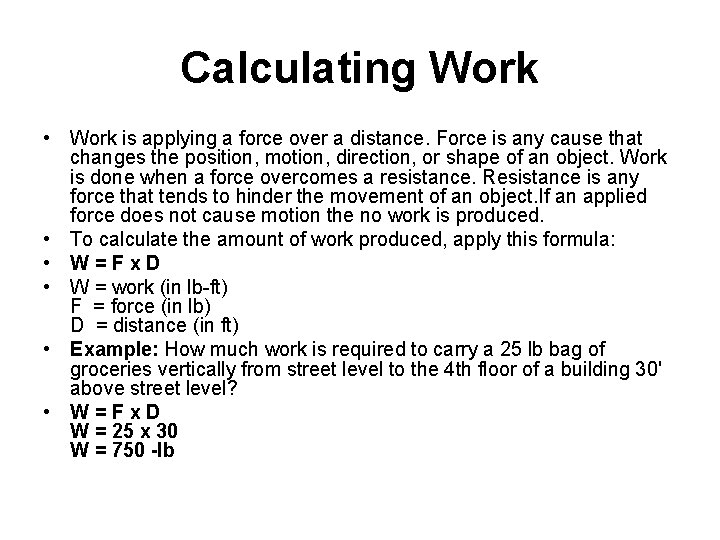Calculating Work • Work is applying a force over a distance. Force is any cause that changes the position, motion, direction, or shape of an object. Work is done when a force overcomes a resistance. Resistance is any force that tends to hinder the movement of an object. If an applied force does not cause motion the no work is produced. • To calculate the amount of work produced, apply this formula: • W = F x D • W = work (in lb-ft) F = force (in lb) D = distance (in ft) • Example: How much work is required to carry a 25 lb bag of groceries vertically from street level to the 4 th floor of a building 30' above street level? • W = F x D W = 25 x 30 W = 750 -lbPneumatic Actuator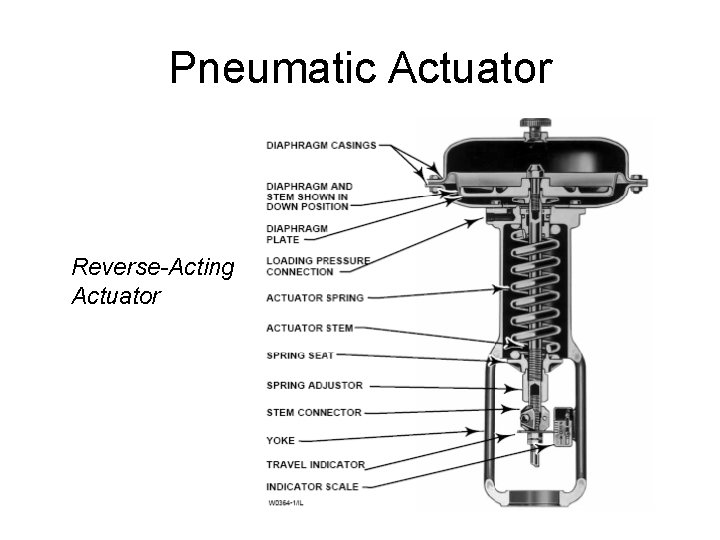Pneumatic Actuator Reverse-Acting Actuator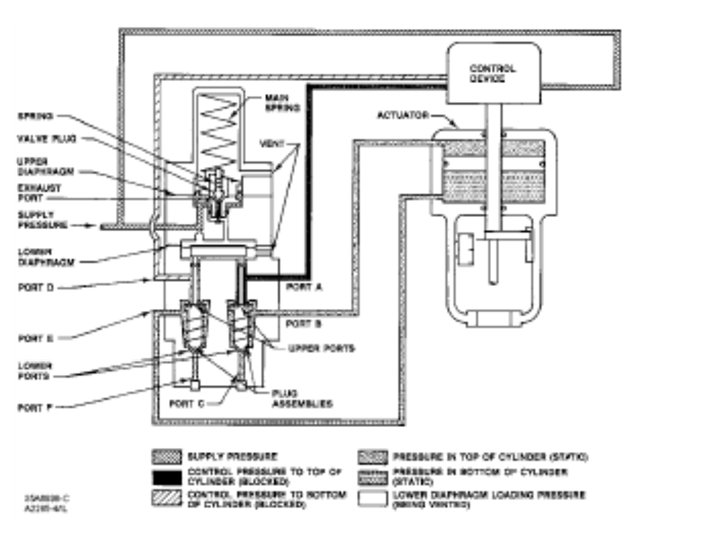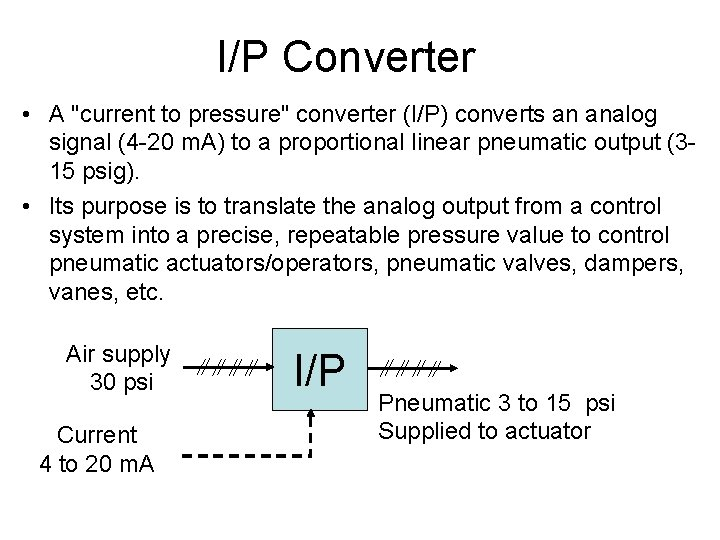I/P Converter • A "current to pressure" converter (I/P) converts an analog signal (4 -20 m. A) to a proportional linear pneumatic output (315 psig). • Its purpose is to translate the analog output from a control system into a precise, repeatable pressure value to control pneumatic actuators/operators, pneumatic valves, dampers, vanes, etc. Air supply 30 psi Current 4 to 20 m. A I/P Pneumatic 3 to 15 psi Supplied to actuator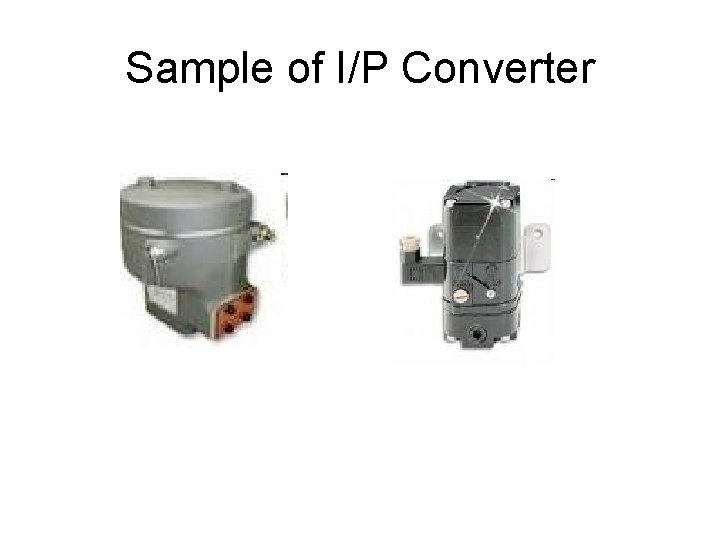Sample of I/P ConverterGeneration and distribution of pneumatic pressure • Compressor is needed for pneumatic system PC PS Regulator valve Tank To I/P compressor 100 psi 30 psiHydraulic Actuator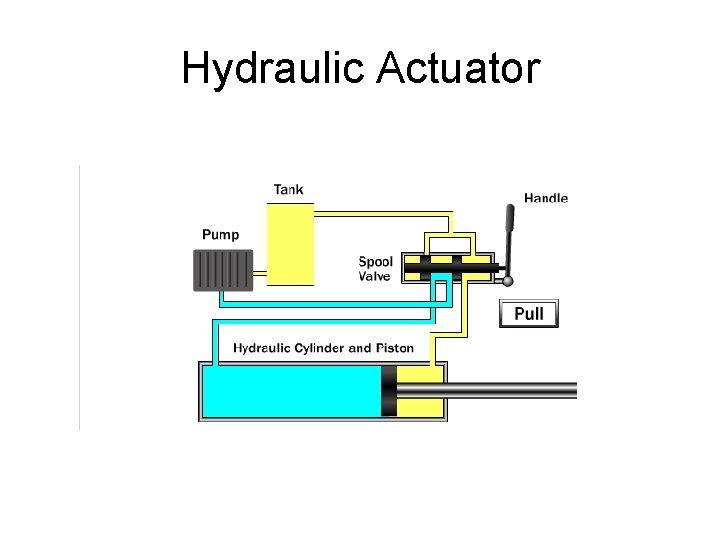Hydraulic Actuator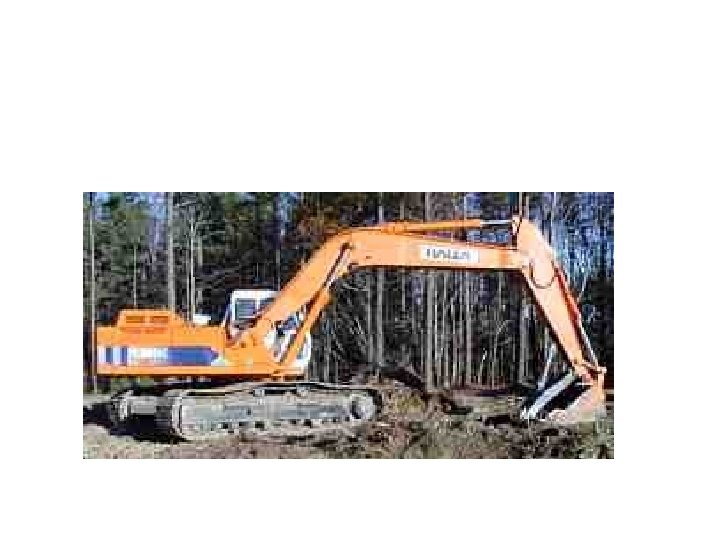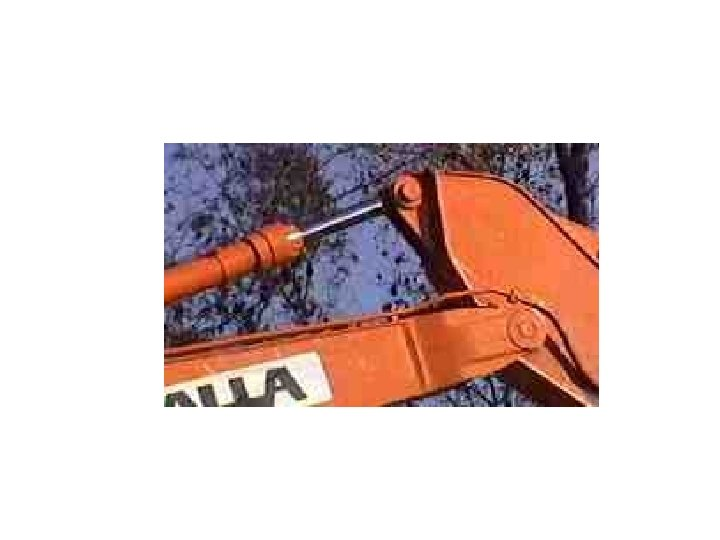Hydraulic Actuator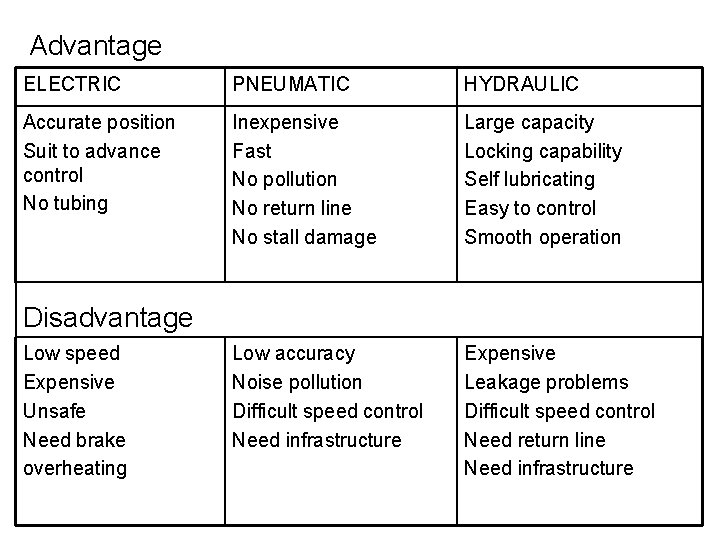Advantage ELECTRIC PNEUMATIC HYDRAULIC Accurate position Suit to advance control No tubing Inexpensive Fast No pollution No return line No stall damage Large capacity Locking capability Self lubricating Easy to control Smooth operation Low accuracy Noise pollution Difficult speed control Need infrastructure Expensive Leakage problems Difficult speed control Need return line Need infrastructure Disadvantage Low speed Expensive Unsafe Need brake overheating